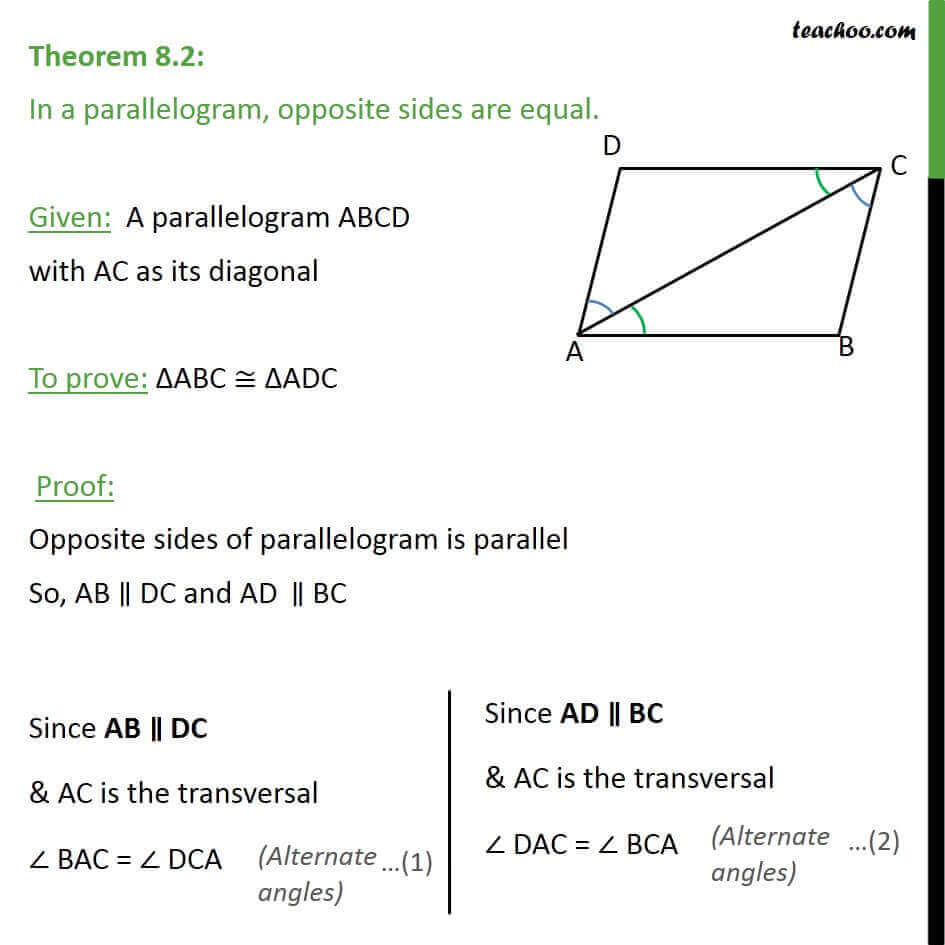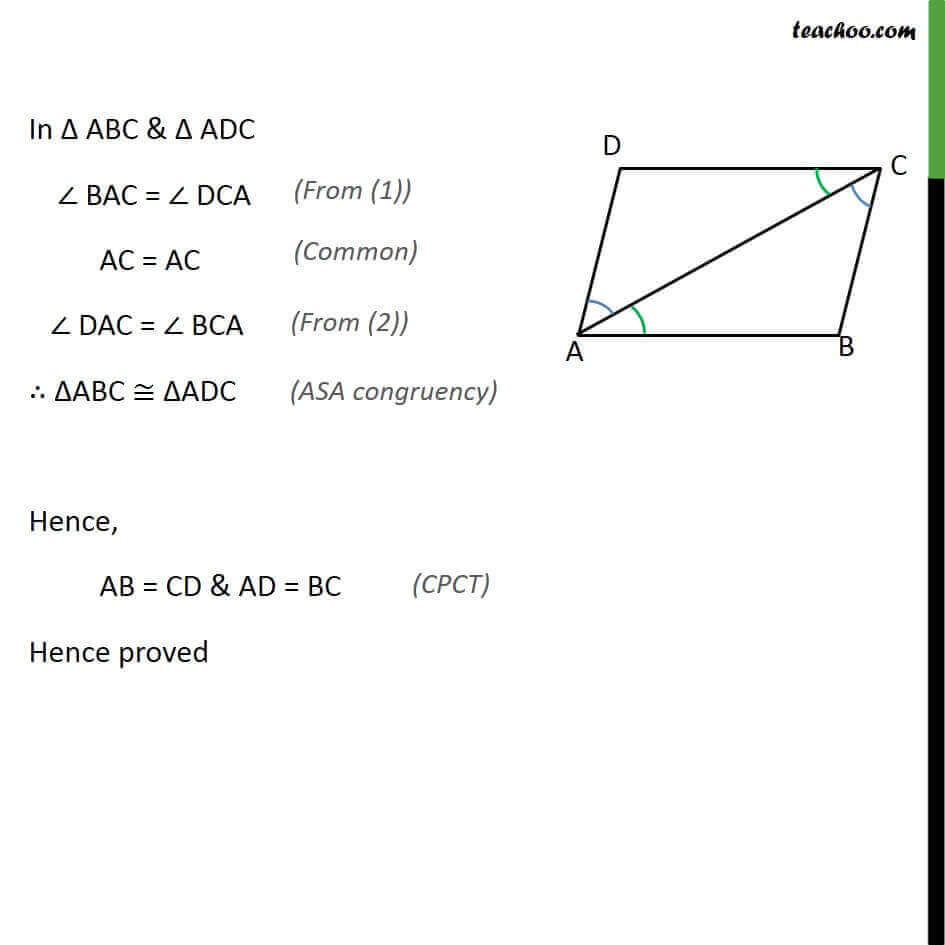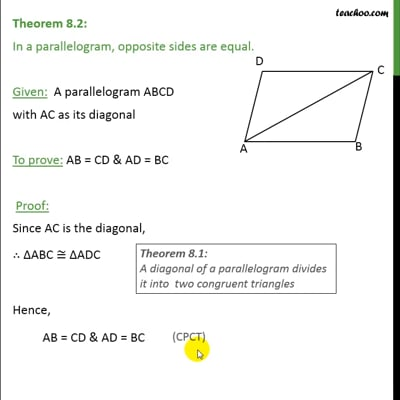Theorems

Chapter 8 Class 9 Quadrilaterals (Term 2)
Serial order wiseThis video is only available for Teachoo black users

### Transcript

Theorem 8.2: In a parallelogram, opposite sides are equal. Given: A parallelogram ABCD with AC as its diagonal To prove: ΔABC ≅ ΔADC Proof: Opposite sides of parallelogram is parallel So, AB ∥ DC and AD‖∥ BC In Δ ABC & Δ ADC ∠ BAC = ∠ DCA AC = AC ∠ DAC = ∠ BCA ∴ ΔABC ≅ ΔADC Hence, AB = CD & AD = BC Hence proved